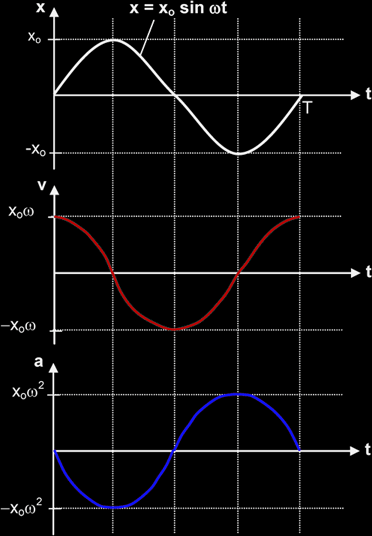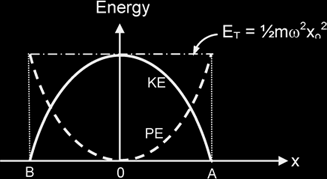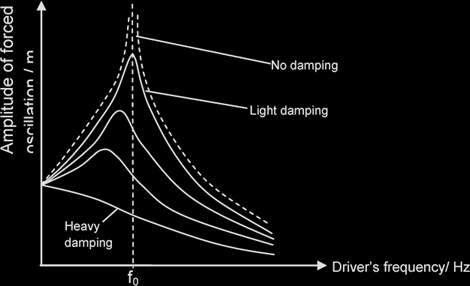# Oscillations

Period: is defined as the time taken for one complete oscillation.

Frequency: is defined as the number of oscillations per unit time,

f = 1 / T

Angular frequency ω: is defined by the eqn, ω = 2 π f. It is thus the rate of change of angular displacement (measured in radians per sec)

Amplitude: The maximum displacement from the equilibrium position.

Phase difference φ: A measure of how much one wave is out of step with another wave, or how much a wave particle is out of phase with another wave particle.

φ = 2πx / λ = t / T x 2π {x =separation in the direction of wave motion between the 2 particles}

Simple harmonic motion: An oscillatory motion in which the acceleration {or restoring force} is

• always proportional to, and
• opposite in direction to the displacement from a certain fixed point / equilibrium position

ie a = -ω2 x (Defining equation of S.H.M)

Time Equations Displacement Equations
x = xo sinωt or x = xo cos (ωt), etc [depending on the initial condition]
v = dx / dt = ωxo cosωt [assuming x = xosinωt] v = ± ω √(xo2 - x2) [v - x graph is an ellipse]
a = -ω2x = -ω2(xosinωt) a = -ω2x
KE = ½ mv2 = ½ m(ωxo cosωt)2 KE = ½ mv2 = ½ mω2 (xo2 - x2) [KE - x graph is a parabola]The energy of the oscillator changes from potential to kinetic and back to potential in every half-cycle interval. At any point of its motion, the sum of the PE and KE is equal to the total energy. At the equilibrium position, the mass has a maximum KE because its speed is greatest and zero PE as the spring neither compressed nor stretched. At either A or B where the mass stops, its KE is zero while its PE is maximum.

The constant interchange of energies during SHM can be represented graphically as follows.It can be shown that

total energy = maximum KE = maximum PE = ½mω2xo2

Damping: refers to the loss of energy from an oscillating system to the environment due to dissipative forces {eg, friction, viscous forces, eddy currents}

Light Damping: The system oscillates about the equilibrium position with decreasing amplitude over a period of time.

Critical Damping: The system does not oscillate & damping is just adequate such that the system returns to its equilibrium position in the shortest possible time.

Heavy Damping: The damping is so great that the displaced object never oscillates but returns to its equilibrium position very very slowly.

Free Oscillation: An oscillating system is said to be undergoing free oscillations if its oscillatory motion is not subjected to an external periodic driving force. The system oscillates at its natural freq.

Forced Oscillation: In contrast to free oscillations, an oscillating system is said to undergo forced oscillations if it is subjected to an input of energy from an external periodic driving force. The freq of the forced {or driven} oscillations will be at the freq of the driving force {called the driving frequency} ie. no longer at its own natural frequency.

Resonance: A phenomenon whereby the amplitude of a system undergoing forced oscillations increases to a maximum. It occurs when the frequency of the periodic driving force is equal to the natural frequency of the system.

#### Effects of Damping on Freq Response of a system undergoing forced oscillations

1. Resonant frequency decreases
2. Sharpness of resonant peak decreases
3. Amplitude of forced oscillation decreases##### Examples of Useful Purposes of Resonance
1. Oscillation of a child's swing.
2. Tuning of musical instruments.